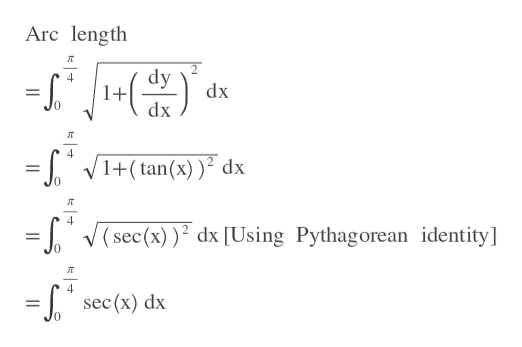# find the exact length of the curve of the equation below, show all worky = ln(sec(x)), with a range of 0 to pi/4

Question
1 views

find the exact length of the curve of the equation below, show all work

y = ln(sec(x)), with a range of 0 to pi/4

check_circle

Step 1

First, we find the dy/dx

Step 2

Then we find arc length using a...help_outlineImage TranscriptioncloseArc length dy 1+ dx 4 dx 4 1+(tan(x) ) dx 0 4 V(sec(x)) dx [Using Pythagorean identity] 4 sec (x) dx 0 fullscreen

### Want to see the full answer?

See Solution

#### Want to see this answer and more?

Solutions are written by subject experts who are available 24/7. Questions are typically answered within 1 hour.*

See Solution
*Response times may vary by subject and question.
Tagged in

### Integration